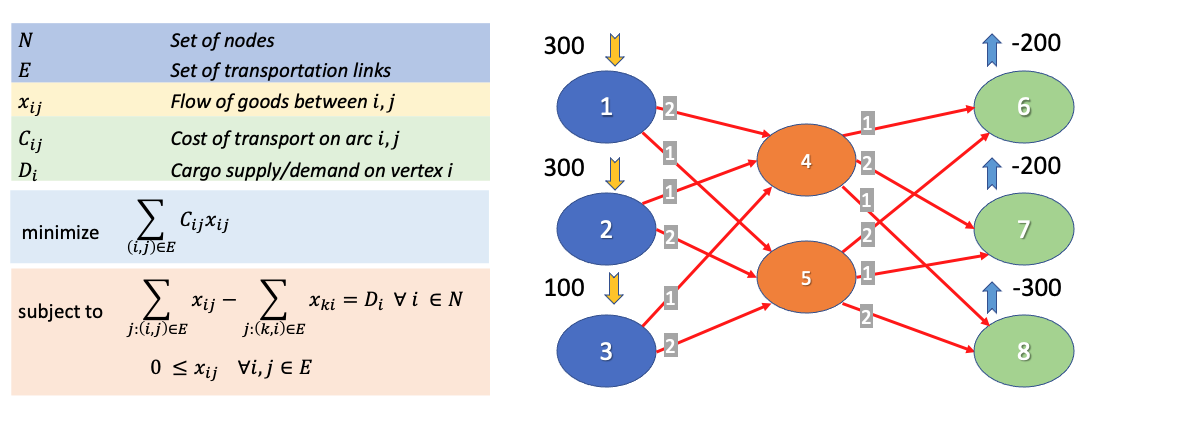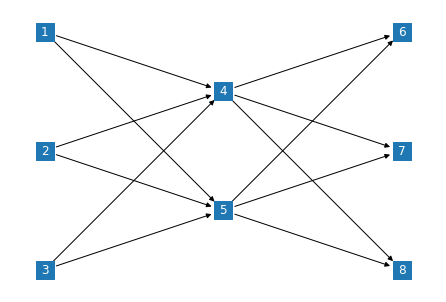# Notebook 3.5 - Minimum Cost Flow using NetworkX and OR-Tools¶

I do appreciate that some PuLP models might look a bit intimidating at first. Do not despair however - the primary purpose of these notebooks is to provide you with an arsenal of examples and templates that you can reuse, when it is time for you to implement your own models. You are not expected to be able to come up with the entire formulations by your own - even in practice (industry, or academia), we always refer to existing models when we build something new.

A very useful resource that every programmer uses these days is a website called StackOverflow - you might have encountered already if you have googled anything related to Python, or programming in general. I would advise that you have a look at the questions related to PuLP, using this link here.

GitHub is another very useful resource, as it currently happens to be the most popular online repository of codes (regardless of language). You might find this link as it points to repositories that contain the keywords "pulp" and "linear".

I did mention before that one of the reasons that we are using Python in this module is the wide variety of options when it comes to existing to the availability of packages that we can use in order to achieve certain tasks.

In this notebook we are going to cover two additional options that we have available at our disposal when it comes to solving minimum cost flow problems.## Solving the MCF using NetworkX¶

As it happens, the networkx package contains several built-in libraries, that we could use in order to solve a range of network flow problems - you can see the full list of algorithms here.

From the above, we can use networkx.algorithms.flow.network_simplex to obtain solutions for simple MCF models (more info here).

As you will see below, the network_simplex algorithm provided by networkx can be a lot more straightforward to use than a full pulp model, as it involves little more than simply declaring the graph.

In :
import networkx as nx
import matplotlib.pyplot as plt

G = nx.DiGraph()



Let us plot the graph, to see what it looks like. We will use the code snippet that we developed last week.

In :
node_pos = {1:(1,3),2:(1,2),3:(1,1),4:(2,2.5),5:(2,1.5),6:(3,3),7:(3,2),8:(3,1)}

nx.draw(G, node_pos, with_labels = True, font_color = 'white', node_shape='s')Obtaining a result is simply a matter of calling:

In :
flowCost, flowDict = nx.network_simplex(G)

print('Minimum cost:', flowCost)
print('')

for key_i, inner_dict in flowDict.items():
for key_j, inner_val in inner_dict.items():
print(f'{key_i}->{key_j} \t Flow: {inner_val}')

Minimum cost: 1500

1->4 	 Flow: 100
1->5 	 Flow: 200
2->4 	 Flow: 300
2->5 	 Flow: 0
3->4 	 Flow: 100
3->5 	 Flow: 0
4->6 	 Flow: 200
4->7 	 Flow: 0
4->8 	 Flow: 300
5->6 	 Flow: 0
5->7 	 Flow: 200
5->8 	 Flow: 0


## Summary¶

import networkx as nx

G = nx.DiGraph()

# SOLUTION
flowCost, flowDict = nx.network_simplex(G)

print('SOLUTION:')
for key_i, inner_dict in flowDict.items():
for key_j, inner_val in inner_dict.items():
print(f'\t\tFlow in link ({key_i},{key_j}) = {inner_val}')

print('\n') # Prints a blank line
print(f'OBJECTIVE VALUE: {flowCost}')


While we can all agree that this code is a lot simpler than our PuLP formulation, please keep in mind that this is as far as we can go, unlike PuLP we do not have the option of adding custom constraints that go beyond the basic MCF formulation.

And of course, NetworkX cannot help us with optimisation problems that do not take place within simple graph structures.

## Solving the MCF using OR-Tools (OPTIONAL)¶

OR-Tools is an optimisation modeller develop by Google, and used extensively by them in-house in order to optimise a very wide range of their operations.

In terms of functionality, it overlaps with PuLP to some extend, as it can be used to implement model formulations, which can be solved either using their internal solver.

However, as you can see in their examples here, the syntax of their models is a lot more tedious to use than PuLP. The reason why we would prefer to use PuLP, is that the syntax of its models does not differ that much from mathematical formulations.

The reason why I mention OR-Tools, however, is because it contains a series of helpful model-specific solvers that can be used to obtain solutions to a range of optimisation problems, without us having to write a formulation.

For a full list of the problem-specific solvers provided by OR-Tools, you can have a look at the list here. The ones that relate to problems that we will encounter in this module are:

In the first instance, we need to make sure that or-tools is installed:

In :
!pip install ortools --progress-bar off

Requirement already satisfied: ortools in /Users/pan/anaconda3/lib/python3.7/site-packages (8.0.8283)
Requirement already satisfied: six>=1.10 in /Users/pan/anaconda3/lib/python3.7/site-packages (from ortools) (1.15.0)
Requirement already satisfied: protobuf>=3.13.0 in /Users/pan/anaconda3/lib/python3.7/site-packages (from ortools) (3.13.0)
Requirement already satisfied: setuptools in /Users/pan/anaconda3/lib/python3.7/site-packages (from protobuf>=3.13.0->ortools) (49.6.0.post20200814)


The OR-Tools MCF modeller requires us to define the network architecture using a series of arrays, each pertaining to the various properties of the links in our graph:In :
start_nodes = [ 1, 1, 2, 2, 3, 3, 4, 4, 4, 5, 5, 5]
end_nodes   = [ 4, 5, 4, 5, 4, 5, 6, 7, 8, 6, 7, 8]
unit_costs  = [ 2, 1, 1, 2, 1, 2, 1, 2, 1, 2, 1, 2]


Our problem instance does not have a given capacity limit for links, and we will therefore use a very large number instead. Unfortunately OR-Tools does not give us the option to define an arc without also providing a capacity.

In :
capacity = 9999999


Similarly, we can provide an array of supply values for each node.

In :
supplies = [300, 300, 100, 0, 0, -200, -200, -300]


We can now implement the model instance:

In :
list(range(1, len(start_nodes)+1))

Out:
[1, 2, 3, 4, 5, 6, 7, 8, 9, 10, 11, 12]
In :
from ortools.graph import pywrapgraph

model = pywrapgraph.SimpleMinCostFlow()

for i in range(len(start_nodes)):

for i in range(len(supplies)):
model.SetNodeSupply(i+1, supplies[i])


The above declaration created 8 node elements in our array, which were generated using an in-line for loop. For every value in a range of integers between 0 and 8, the str(i+1) command was adding a stringified version of the value, incremented by one.

The increment is essential if we want the list of node names to start from 1, since the range(8) command would return a series of 8 values, starting from 0. You can check this on your own below:

In :
model.Solve()

print('Minimum cost:', model.OptimalCost())
print('')
for i in range(model.NumArcs()):

Minimum cost: 1500

1->4 	 Flow: 0
1->5 	 Flow: 300
2->4 	 Flow: 300
2->5 	 Flow: 0
3->4 	 Flow: 100
3->5 	 Flow: 0
4->6 	 Flow: 200
4->7 	 Flow: 0
4->8 	 Flow: 200
5->6 	 Flow: 0
5->7 	 Flow: 200
5->8 	 Flow: 100


## Summary¶

As you can see below, this is the most compact MCF formulation that we managed to implement so far!

from ortools.graph import pywrapgraph

start_nodes = [ 1, 1, 2, 2, 3, 3, 4, 4, 4, 5, 5, 5]
end_nodes   = [ 4, 5, 4, 5, 4, 5, 6, 7, 8, 6, 7, 8]
unit_costs  = [ 2, 1, 1, 2, 1, 2, 1, 2, 1, 2, 1, 2]
capacity    = 9999999
supplies    = [300, 300, 100, 0, 0, -200, -200, -300]

model = pywrapgraph.SimpleMinCostFlow()